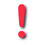# Introduction & notation(If you are experiencing problems with the display of the mathematical formula, you can either try to use another browser, or use this link which should work smoothly: http://popix.lixoft.net)

Models are attempts to describe observations in a logical, simple way, involving the relationship between measurements, parameters, covariates and so on. If working in a probabilistic framework - as we are here - there will be randomness in the model, involving random variables, probability distributions, errors and more.

Because of this, we are going to make the following definition of a model in this context: a model is a joint probability distribution.

Therefore, defining a model means defining a joint probability distribution, which can then be decomposed into a product of conditional distributions we can perform tasks on: estimation, model selection, simulation, etc.

This chapter is therefore about defining appropriate probability distributions. We start by introducing some general notation and conventions.

• We will call $y_i$ the set of observations recorded on subject $i$, and $\by$ the combined set of observations for all the $N$ individuals: $\by = (y_1, ...,y_N)$. In general, we will use bold text (like for $\by$) when a variable regroups several individuals. Thus, we write $\psi_i$ for the parameter vector for individual $i$ and $\bpsi$ the parameter vector of a set of individuals, $\bpsi = (\psi_1,\ldots,\psi_N)$.

• We note $\qy$ and $\qpsi$ the distributions of $\by$ and $\bpsi$ respectively, $\qcypsi$ the conditional distribution of $\by$ given $\bpsi$, and $\qypsi$ the joint distribution of $\by$ and $\bpsi$. In these (and other distributions), we have placed the variable described by the distribution in the index.

• When there is no ambiguity when working with whole equations, to simplify notation we may omit the indices and simply use the symbol $\pmacro$. For instance, $\qy(\by)$, the pdf of $\by$, becomes $\py(\by)$; both are equivalent. The symbol $\pmacro$ has no meaning on its own, it is completely defined by its arguments.

• When the distribution of the individual parameters $\psi_i$ of subject $i$ depends on a vector of individual covariates $c_i$ and a population parameter $\theta$, we may choose to explicitly show this dependence by writing the distribution of $\psi_i$ as $\ppsii(\psi_i;c_i,\theta)$.

• When the conditional distribution $\qcyipsii$ of the observations $y_i=(y_{ij}, 1\leq j \leq n_i)$ of individual $i$ depends on regression variables $x_i=(x_{ij}, 1\leq j \leq n_i)$ and source terms $u_i$, (i.e., inputs of a dynamical system such as doses in a pharmacokinetic model), we may choose to explicitly show this dependence, writing the conditional distribution as $\pcyipsii(y_i | \psi_i;x_i,u_i)$.

There are two important pieces to the puzzle: the observations $\by$ whose distribution $\qy$ depends on the individual parameters, and the individual parameters $\bpsi$ themselves with distribution $\qpsi$. In the population approach, the base distribution is the joint distribution $\qypsi$ of the observations and individual parameters:

$$\pypsi(\by,\bpsi) = \pcypsi(\by | \bpsi)\ppsi(\bpsi).$$

In this chapter, we concentrate essentially on these two components: the conditional distribution $\qcypsi$ of the observations, and the distribution $\qpsi$ of the individual parameters.

Depending on the required complexity of the model, its other components such as covariates, population parameters and design can also be modeled as random variables, but we will not go into such detail in this chapter.

For each model, we aim to precisely identify the minimal amount of information needed to represent it mathematically, so that it remains possible to implement and analyze. To do this, we will be able to use $\mlxtran$, a powerful formal declarative language that allows us to describe complicated structural and statistical models in a straightforward, intuitive way.